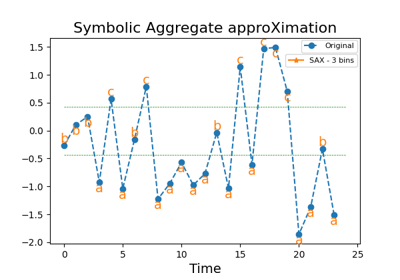# `pyts.approximation`.SymbolicAggregateApproximation¶

class `pyts.approximation.``SymbolicAggregateApproximation`(n_bins=4, strategy='quantile', raise_warning=True, alphabet=None)[source]

Symbolic Aggregate approXimation.

Parameters: n_bins : int (default = 4) The number of bins to produce. It must be between 2 and `min(n_timestamps, 26)`. strategy : ‘uniform’, ‘quantile’ or ‘normal’ (default = ‘quantile’) Strategy used to define the widths of the bins: ‘uniform’: All bins in each sample have identical widths ‘quantile’: All bins in each sample have the same number of points ‘normal’: Bin edges are quantiles from a standard normal distribution raise_warning : bool (default = True) If True, a warning is raised when the number of bins is smaller for at least one sample. In this case, you should consider decreasing the number of bins or removing these samples. alphabet : None, ‘ordinal’ or array-like, shape = (n_bins,) Alphabet to use. If None, the first n_bins letters of the Latin alphabet are used. If ‘ordinal’, integers are used.

References

  J. Lin, E. Keogh, L. Wei, and S. Lonardi, “Experiencing SAX: a novel symbolic representation of time series”. Data Mining and Knowledge Discovery, 15(2), 107-144 (2007).

Examples

```>>> from pyts.approximation import SymbolicAggregateApproximation
>>> X = [[0, 4, 2, 1, 7, 6, 3, 5],
...      [2, 5, 4, 5, 3, 4, 2, 3]]
>>> transformer = SymbolicAggregateApproximation()
>>> print(transformer.transform(X))
[['a' 'c' 'b' 'a' 'd' 'd' 'b' 'c']
['a' 'd' 'c' 'd' 'b' 'c' 'a' 'b']]
```

Methods

 `__init__`([n_bins, strategy, raise_warning, …]) Initialize self. `fit`([X, y]) Pass. `fit_transform`(X[, y]) Fit to data, then transform it. `get_params`([deep]) Get parameters for this estimator. `set_params`(**params) Set the parameters of this estimator. `transform`(X) Bin the data with the given alphabet.
`__init__`(n_bins=4, strategy='quantile', raise_warning=True, alphabet=None)[source]

Initialize self. See help(type(self)) for accurate signature.

`fit`(X=None, y=None)[source]

Pass.

Parameters: X Ignored y Ignored
`fit_transform`(X, y=None, **fit_params)

Fit to data, then transform it.

Fits transformer to X and y with optional parameters fit_params and returns a transformed version of X.

Parameters: X : array-like, shape = (n_samples, n_timestamps) Univariate time series. y : None or array-like, shape = (n_samples,) (default = None) Target values (None for unsupervised transformations). **fit_params : dict Additional fit parameters. X_new : array Transformed array.
`get_params`(deep=True)

Get parameters for this estimator.

Parameters: deep : bool, default=True If True, will return the parameters for this estimator and contained subobjects that are estimators. params : dict Parameter names mapped to their values.
`set_params`(**params)

Set the parameters of this estimator.

The method works on simple estimators as well as on nested objects (such as `Pipeline`). The latter have parameters of the form `<component>__<parameter>` so that it’s possible to update each component of a nested object.

Parameters: **params : dict Estimator parameters. self : estimator instance Estimator instance.
`transform`(X)[source]

Bin the data with the given alphabet.

Parameters: X : array-like, shape = (n_samples, n_timestamps) Data to transform. X_new : array, shape = (n_samples, n_timestamps) Binned data.

## Examples using `pyts.approximation.SymbolicAggregateApproximation`¶Symbolic Aggregate approXimation